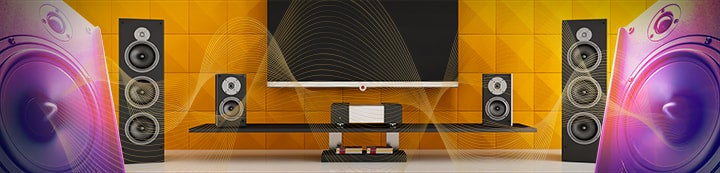1 - 10 of 10 Posts

#### mark_anderson_us

·
##### Registered
Joined
·
157 Posts
Discussion Starter · ·
Hi All

This is a bit off topic, but I figured one of the experts might be able to answer this (just getting started with REW by the way--waiting for Galaxy to arrive--looks like a great program)

I'm working on a project where I want to express the sound proofing effect of a fabric (in simplistic terms) in percentage for very non-technical readers (they've never even heard of DB). So my plan is to put my SPL in an enclosure with one open side facing a speaker, generate a test signal and measure the SPL. Then cover the enclosure with the fabric and repeat the test.

I recall a few facts from some of my electronics studies many years ago:
• DB is a logarithmic scale
• -3db is half power

So (with real simple numbers) let's assume I measure a 1khz signal without the fabric and the SPL is 80db, I then measure it with the fabric and the level is 75db, so a difference of -5. How would I express that as an approximate % difference?

Regards

Mark

#### JohnM

·
Joined
·
6,711 Posts
To use power ratios, the proportion is 10 to the power of (dB/10), so e.g. 10 to the power of (-3/10) is 0.501, or 50.1%. For -5dB the figure is 10^(-5/10) = 0.316, or 31.6%.

#### mark_anderson_us

·
##### Registered
Joined
·
157 Posts
Discussion Starter · ·
Thanks!

·
Joined
·
239 Posts

#### wbz

·
Joined
·
22 Posts
Nice, thanks for that.

#### mark_anderson_us

·
##### Registered
Joined
·
157 Posts
Discussion Starter · ·
Here is little online calculator for THD as percent to dB and dB to percent:

it is based on formula: dB = -20 *log(v1/v2), thus -6dB is roughly 50%.

Andrew
Thanks Andrew

So if I'm reading that chart right -40 DB is 100th

thus if source 1 was 75DB and source 2 was 35DB, source 1 would be 100x louder than source 2

#### Barleywater

·
##### Registered
Joined
·
239 Posts
All else being equal, louder source would have 100x drive level. Perceived loudness is different. Doubling drive level, 6.02dB quadruples power, but takes closer to 10dB to get perceived doubling in loudness (as I recall).

Andrew

#### Ed Mullen

·
Joined
·
392 Posts
Don't confuse the change in acoustic sound pressure (in Pascals) with perceived loudness curves (more on that below).

Once dB is converted to Pascals (which is a linear function), the percent change in acoustic sound pressure can be determined.

Baseline = starting sound pressure level.
+1 dB = 1.12X baseline
+2 dB = 1.26X baseline
+3 dB = 1.41X baseline
+4 dB = 1.58X baseline
+5 dB = 1.78X baseline
+6 dB = 2X baseline
+7 dB = 2.24X baseline
+8 dB = 2.51X baseline
+9 dB = 2.82X baseline
+10 dB = 3.16X baseline
+11 dB = 3.55X baseline
+12 dB = 4X baseline

Say for example you have one subwoofer being measured outdoors and the SPL at 30 Hz is 90 dB. If you took a second identical subwoofer and place it next to the first one, and also set that subwoofer to 90 dB at 30 Hz, and then turned them both on, they resulting SPL would be 96 dB. So a 6 dB increase in SPL is literally a doubling of the acoustic output. This can be verified by converting to 90 dB to Pascals and 96 dB to Pascals and you will note the 96 dB Pascal value is literally 2X the 90 dB Pascal value. Similarly, if you took two more subwoofers (for a total of four subwoofers), all operating individually at 90 dB @ 30 Hz, the combined SPL would become 102 dB. Again converting to Pascals, 102 dB is 4X more than 90 dB in Pascals.

If you take a look at the equal loudness curves, the old '10 dB = a doubling of perceived loudness' only hold true over certain portions of the audible spectrum, and equal loudness curves are expressed differently than actual changes in SPL, so don't confuse them - more on that below.

You'll note that the equal loudness curves compress in the deep bass regions. So while we're less sensitive to deep bass frequencies (meaning the hearing threshold is higher), once that threshold is exceeded, wer'e actually much more sensitive to perceived loudness changes than we are at higher frequencies.

Finally note the equal loudness curves are expressed in sound intensity and not sound pressure, and the two units are not the same (the log10 functions are different), despite the fact they are both dB scales.

http://hyperphysics.phy-astr.gsu.edu/hbase/sound/eqloud.html

#### mark_anderson_us

·
##### Registered
Joined
·
157 Posts
Discussion Starter · ·
Thanks!

1 - 10 of 10 Posts